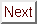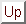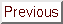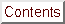Next: Experimental Results: Temperature Up: Experimental Results: Angular Previous: Tinkham's Formula Fits

## Microscopic Models of Superconductivity and Hc2(theta) in C4KHg

In the following sections on the temperature dependence of the upper critical field, the theories of choice for explaining the data are those which include anisotropic gap or multiband effects. Logical consistency demands that these effects also be considered in attempts to explain the anomalies in Hc2(theta).

Many theorists have calculated the effects of Fermi surface anisotropy, gap anisotropy, or multiband contributions to superconductivity on Hc2(T).[274,33,80,5] Unfortunately, most of these theorists have confined their calculations to one or two high-symmetry directions, so that a great deal of work remains to extract Hc2(theta) from their equations.

The only model to explicitly consider the case of a general field orientation is that developed by K. Takanaka. This model incorporates both Fermi surface anisotropy and gap anisotropy, and is discussed further in Section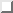. Takanaka gives a full expression for Hc2(theta, T) which could be used to fit the Hc2(theta) data. Takanaka's model was withdrawn, though, because of the unphysical divergence of Hc2(t) below about t = 0.7. While Youngner and Klemm calculated a corrected Hc2(T) that utilized some of Takanaka's ideas, they did not produce an improved Hc2(theta) equation.

Despite the unphysical behavior of Takanaka's model at low temperatures, one might consider fitting it to the experimental data at high reduced temperatures. Takanaka does show Hc2(theta) curves in Ref.  for t = 0.9 which incorporate gap anisotropy effects. Toyota et al. fit their NbSe2 data to the Takanaka equation, but it is not obvious that the Takanaka curve describes their data better than the AGL theory. Furthermore, if anisotropy-induced anomalies are responsible for the deviations of the C4KHg Hc2(theta) data from the AGL model, one would expect the deviations to be greatest at the lowest reduced temperatures. Figureshows that the deviations are in fact greater at higher reduced temperatures, where the simple AGL model should be most applicable. The reason is that the assumptions that go into Ginzburg-Landau theories are justifiable only near Tc, so if a more microscopic model is needed to fit the data, it should describe low-temperature anomalies. To put the matter into common-sense terms, one would expect anisotropy-derived deviations in Hc2(theta) in the same temperature range where the anisotropy is causing anomalies in Hc2(t), i.e., at low reduced temperatures. The larger deviations from the AGL theory at higher reduced temperatures is therefore in conflict with expectations from anisotropic-gap and multiband models, but consistent with type I behavior for small theta, as discussed in Section.

The general problem with trying to fit more microscopic models to the Hc2(theta) curves is that many parameters (tilt, mosaic spread, Hc, epsilon, Hc2(0°)) have already been identified as being relevant. 5 parameters is already a large number for slightly noisy curves of about 40 or 50 points. Considering that the situation is somewhat simpler with the Hc2(T) data, it is hardly surprising that not many detailed theories of H2(theta) have appeared.

Since the Hc2(theta) curves deviate from the AGL theory near Tc and the Hc2(T) curves deviate from the AGL theory near T=0, it does not seem too far-fetched to attribute different causes to these anomalies. With this decoupling in mind, the most convincing explanation for the shape of the Hc2(theta) curves in C4KHg would seem to be that based on type I superconductivity near vecH || ^c. This explanation is embodied by Eqn., which allows for type I behavior. The anomalies in Hc2(T) are discussed in the section that follows.Next: Experimental Results: Temperature Up: Experimental Results: Angular Previous: Tinkham's Formula Fits

alchaiken@gmail.com (Alison Chaiken)
Wed Oct 11 22:59:57 PDT 1995